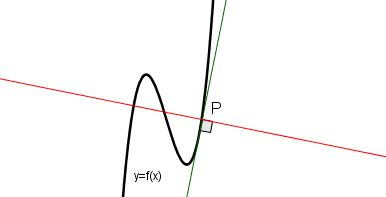Calculus

# Normal to a Curve

Below is the graph of the curve $y=f(x).$ The green line is tangential to the curve at the point $P=(a,f(a)).$ If the red line is perpendicular to the green line and the equation of the red line is $y=-\frac{1}{5}x+15,$ what is the value of $f'(a)?$What is the normal to the curve $y=6\tan x + 4$ at the point $\left(\frac{\pi}{4}, 10\right)$?

What is the equation of the line $l$ which passes through the point $(5,2)$ and is perpendicular to the line passing through the two points $(3,3)$ and $(9,4)?$

What is the normal to the curve $y=2x^2 - 3x -15$ at the point $(3, -6)$?

What is the normal to the curve $y=10{e}^x + {x}^2 +5$ at the point $(3,10{e}^3+14 )$?

×